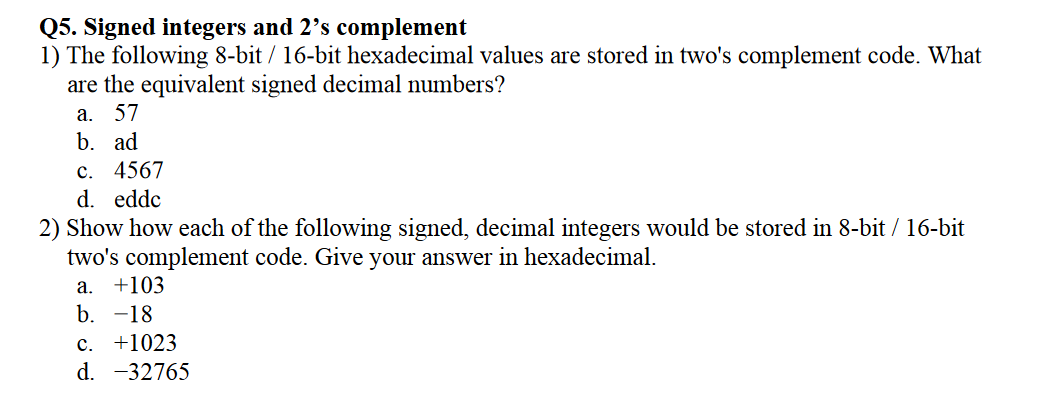# Question Solved1 AnswerQ5. Signed integers and 2's complement 1) The following 8-bit/16-bit hexadecimal values are stored in two's complement code. What are the equivalent signed decimal numbers? a. 57 b. ad c. 4567 d. eddc 2) Show how each of the following signed, decimal integers would be stored in 8-bit / 16-bit two's complement code. Give your answer in hexadecimal. +103 b. -18 c. +1023 d. -32765 a.OEEBGI The Asker · Computer ScienceTranscribed Image Text: Q5. Signed integers and 2's complement 1) The following 8-bit/16-bit hexadecimal values are stored in two's complement code. What are the equivalent signed decimal numbers? a. 57 b. ad c. 4567 d. eddc 2) Show how each of the following signed, decimal integers would be stored in 8-bit / 16-bit two's complement code. Give your answer in hexadecimal. +103 b. -18 c. +1023 d. -32765 a.
More
Transcribed Image Text: Q5. Signed integers and 2's complement 1) The following 8-bit/16-bit hexadecimal values are stored in two's complement code. What are the equivalent signed decimal numbers? a. 57 b. ad c. 4567 d. eddc 2) Show how each of the following signed, decimal integers would be stored in 8-bit / 16-bit two's complement code. Give your answer in hexadecimal. +103 b. -18 c. +1023 d. -32765 a.